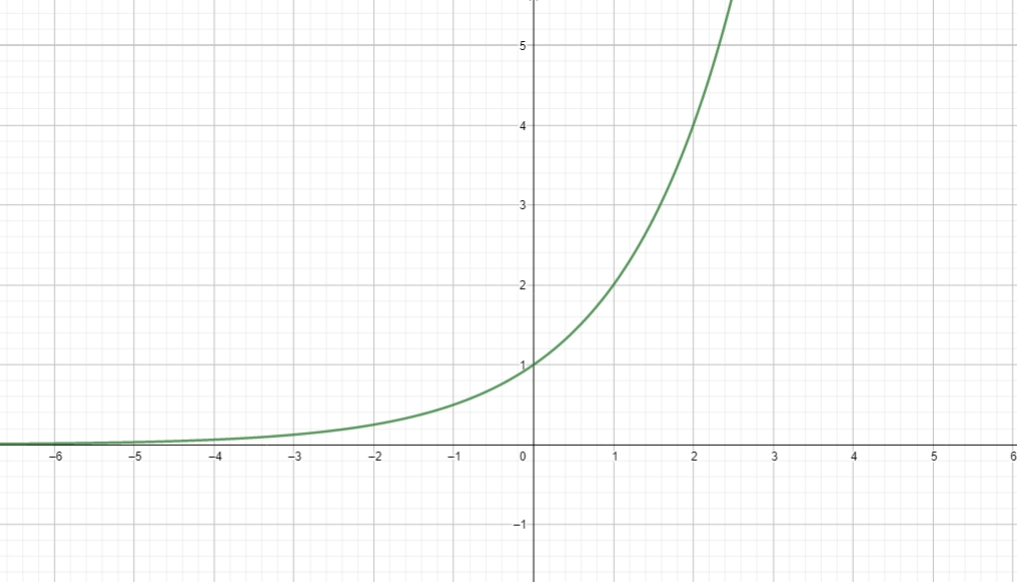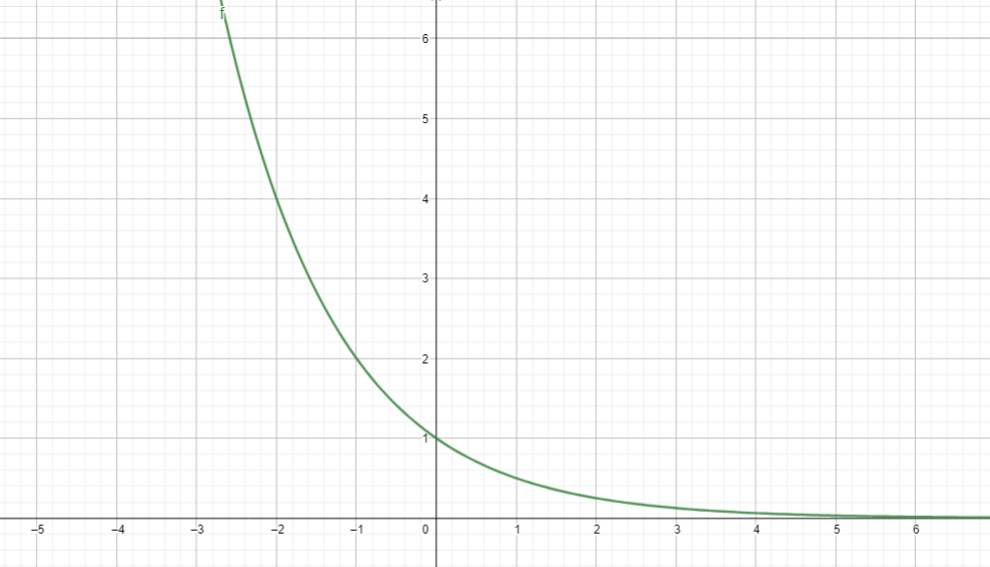407 493 6601

# Algebra 1 - Exponential Functions

## Introduction

• The exponential function is a function in the form of .
• The domain of $\mathbit{f}$ is the set of all real numbers, and the range is the set of all positive real numbers.
• The line $\mathbit{y}\mathbf{=}\mathbf{0}$ (x-axis) is the horizontal asymptote of $\mathbit{f}\left(x\right)\mathbf{=}{\mathbit{b}}^{\mathbf{x}}$.
• If the function is increasing, it is in the form
• If the function is decreasing, it is in the form of .
• The graph of  is shown below:• The graph of  is shown below:## Natural Exponential Function, Exponential Growth, and Exponential Decay

• Natural Exponential Function is a function in the form of .
• Euler's number is an irrational number that is approximately equal to 2.71828 or 2.72.
• This function has the same form of graph and properties as those with .
• The inverse of the natural exponential function is the natural logarithmic function.

Exponential Growth

• A mathematical model of population increase is given by $y={y}_{0}{\left(e\right)}^{rt}$ , where $y$ is the population after $t$ years, ${y}_{0}$ is the present population, and $r$ is the population growth rate.
• For the doubling time growth model, the equation is $y={y}_{0}{\left(2\right)}^{\frac{t}{T}}$, where ${y}_{0}$ is the present population, $y$ is the population after $t$ years, $T$ is the doubling, and $t$ is the time in years.
• Another form of exponential growth is the concept of compound interest given by the formula $y={y}_{0}{\left(1+\frac{r}{k}\right)}^{kt}$ where ${y}_{0}$ is the initial amount, $y$ is the value at the end of $t$ years, $r$ is the nominal rate, and $k$ is the number of conversions.
• The appreciation formula is given by $y={y}_{0}{\left(1+r\right)}^{t}$ where $y$ is the increased value after $t$ years, ${y}_{0}$ is the initial value, and $r$ is the rate of increase.
• The expression  is called the growth factor.

Exponential Decay

• This is modeled by the equation $y={y}_{0}{\left(e\right)}^{-rt}$ where $r$ is the rate of decrease, $e$ is Euler's number.
• The half-life decay model is given by $y={y}_{0}{\left(\frac{1}{2}\right)}^{\frac{t}{T}}$ where $T$ is the half-life.
• The formula for depreciation is given by $y={y}_{0}{\left(1-r\right)}^{t}$ where $r$ is the annual rate of depreciation.
• The expression $\left(1-r\right)$ is called the decay factor.

## Transformation of the Graph of Exponential Function

• Transformation of the graph of an exponential function describes its relationship with the parent function $\mathbit{y}\mathbf{=}{\mathbit{b}}^{\mathbf{x}}$
• Reflection: The graph of $\mathbit{y}\mathbf{=}\mathbf{-}\mathbit{f}\left(x\right)$ is the reflection about the x-axis of the graph of $\mathbit{y}\mathbf{=}\mathbit{f}\left(x\right)$. The graph of $\mathbit{y}\mathbf{=}\mathbit{f}\left(-x\right)$ is the reflection of the y-axis of the graph of $\mathbit{y}\mathbf{=}\mathbit{f}\left(x\right)$.
• Stretching and Shrinking: In function  the effect can be observed in the graph of the parent function $\mathbit{f}\left(x\right)\mathbf{=}{\mathbit{b}}^{\mathbf{x}}$. If $\mathbit{a}\mathbf{>}\mathbf{1}$, the graph shrinks. If $\mathbf{0}\mathbf{<}\mathbit{a}\mathbf{<}\mathbf{1}$, then the graph of $\mathbit{f}\left(x\right)\mathbf{=}\mathbit{a}{\mathbit{b}}^{\mathbf{x}}$ is wider.

Vertical and Horizontal Shift:

• For function $\mathbit{f}\left(x\right)\mathbf{=}{\mathbit{b}}^{\mathbf{x}\mathbf{-}\mathbf{c}}$, the graph of the parent function $\mathbit{f}\left(x\right)\mathbf{=}{\mathbit{b}}^{\mathbf{x}}$ shifts c units to the right since $\mathbit{c}\mathbf{>}\mathbf{0}\mathbf{.}$
• For function , the graph of the parent function $\mathbit{f}\left(x\right)\mathbf{=}{\mathbit{b}}^{\mathbf{x}}$ shifts c units to the left since $\mathbit{c}\mathbf{<}\mathbf{0}$.
• For function $\mathbit{f}\left(x\right)\mathbf{=}{\mathbit{b}}^{\mathbf{x}}\mathbf{-}\mathbit{d}$, the graph of the parent function shifts d units downward since $\mathbit{d}\mathbf{<}\mathbf{0}\mathbf{.}$ The horizontal asymptote is the line $\mathbit{y}\mathbf{=}\mathbf{-}\mathbit{d}$.
• For function $\mathbit{f}\left(x\right)\mathbf{=}{\mathbit{b}}^{\mathbf{x}}\mathbf{+}\mathbit{d}$, the graph of the parent function shifts d units upward since $\mathbit{d}\mathbf{>}\mathbf{0}$. The horizontal asymptote is the line $\mathbit{y}\mathbf{=}\mathbit{d}$.

## Exponential Equations and Some Solved Examples

• An exponential equation is an equation involving exponential expressions. The following are some examples. Notice that the exponent has variables.
• ${2}^{x}=8$
• ${5}^{2x-3}=625$
• ${\left(\frac{1}{7}\right)}^{x}={343}^{2x-1}$
• Use the One-to-One Property of Exponential Functions in solving exponential equations. Let $b$ be a positive real number, with $b\ne 1$ and let $x$ and $y$ be real numbers. If ${b}^{x}={b}^{y}$, then $x=y.$

Example 1. Solve for $x$ in the equation ${2}^{x}=64$.

Solution:

${2}^{x}=64\to {2}^{x}={2}^{6}$

Thus, we get $x=6$.

Example 2. Solve the equation ${\left(\frac{1}{2}\right)}^{x+7}={\left({64}^{-3}\right)}^{5-x}$

Solution:

${\left(\frac{1}{2}\right)}^{x+7}={\left({64}^{-3}\right)}^{5-x}$

${2}^{-1×\left(x+7\right)}={2}^{6×-3\left(5-x\right)}$

Since there are common bases, we have:

$-1×\left(x+7\right)=6×-3\left(5-x\right)$

$-x-7=-90+18x$

$-7+90=18x+x$

$83=19x$

$x=\frac{83}{19}$

## Solved Examples of Exponential Growth and Exponential Decay

Example 1. The population of a particular city in Florida increases according to the exponential model $y=4500{e}^{0.015t}$ where $t$ is in the years. What will the population be after seven years?

Solution:

$y=4500{e}^{0.015×7}$

Hence, it is predicted to have a total population of 5,000 after seven years.

Example 2. A toxic radioactive substance, Plutonium-230, has a half-life of 24,100 years. Suppose 5 mg of Plutonium was released in a nuclear accident; how much of the 5 mg will remain after 100 years?

Solution:

Use the exponential decay model, $y={y}_{0}{\left(\frac{1}{2}\right)}^{\frac{t}{T}}$

$y=5×{\left(\frac{1}{2}\right)}^{\frac{100}{24100}}$

$y\approx 4.985$ mg

Hence, about 4.985 mg will be left after 100 years.

## Cheat Sheet

• There are two major forms of exponential functions, $f\left(x\right)={b}^{x}$ where  (increasing) and $f\left(x\right)={b}^{x}$ where $0 (decreasing).
• The graphs of the exponential functions $f\left(x\right)={2}^{x}$ and $g\left(x\right)={2}^{-x}$ show a reflection in the y-axis.
• The graphs of the exponential functions $f\left(x\right)={2}^{x}$ and $g\left(x\right)=-{2}^{x}$ show a reflection in the x-axis.
• The horizontal asymptote of the exponential function in the form of $f\left(x\right)={b}^{x}$ is the line $y=0$ (x-axis).
• The horizontal asymptote of $y={2}^{x-3}+1$ is the line $y=1$.
• Using the one-to-one property of exponential functions implies that both expressions must have the same base to solve the equation.

## Blunder Areas

• The base $b$ of an exponential function $f\left(x\right)={b}^{x}$ is always positive. Don't be confused with the form $f\left(x\right)=-{b}^{x}$.
• An exponential function in the forms $f\left(x\right)={b}^{x}$ or $g\left(x\right)={b}^{-x}$ have no x-intercepts. However, the case of $f\left(x\right)={b}^{x}-d$ has an x-intercept.
• Always use the One-to-One Property of Exponential functions in solving exponential equations.
• In the case of exponential equations wherein we cannot change both sides to similar bases, logarithms are applicable. Some examples of these equations are ${7}^{2x-3}=18$${4}^{3+4x}={9}^{2x+1}$, or $15={2}^{8-3x}$.